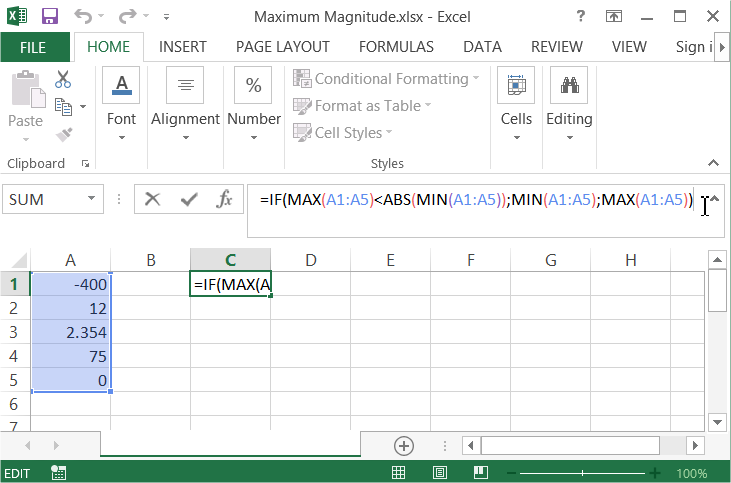## Finding The Maximum Magnitude

In this Excel tutorial you will teach yourself how to find the largest number whether negative or positive out of a range of values. This is not obvious, but you need just one formula.

Just one function is enough to determine the largest magnitude of a subset of values in Excel’s table. You should use below formula:

=IF(MAX(A1:A100)<ABS(MIN(A1:A100));MIN(A1:A100);MAX(A1:A100))Here is the explanation of this formula:First check IF the MAXIMUM value in the A1:A100 range is lower than ABSOLUTE of MINIMUM value. IF it is true that means that the maximum magnitude is a MINIMUM number. Otherwise it is a MAXIMUM number. Remember that a maximum magnitude can be negative or positive number.Here is the whole secret about finding the the maximum magnitude using Microsoft Excel application.

## Template

```Further reading: